#### 期刊菜单

Design of Simple DC Electronic Load Based on STM32
DOI: 10.12677/SEA.2022.113060, PDF, HTML, XML, 下载: 124  浏览: 299

Abstract: This system adopts STM32 microcontroller as the core and uses PWM modulation method to realize simple DC electronic load by using series type dual half-bridge DC-DC circuit as the main circuit of simple electronic load. Based on the current and voltage sampling feedback obtained from the measurement circuit, the microcontroller uses the PID algorithm to adjust the PWM wave duty cycle and control the IR2103 driver circuit output to realize the electronic load to achieve constant current and constant resistance mode stability. The system measures and displays the relevant status of the circuit via OLED in real time. After several experimental tests, this system can stabilize the adjustable current of 100~2000 mA in constant current mode and the adjustable resistance of 10~100 Ω in constant resistance mode, and all the errors of the system are less than 1%, which can be well applied to power supply test equipment.

1. 引言

2. 简易直流电子负载总体设计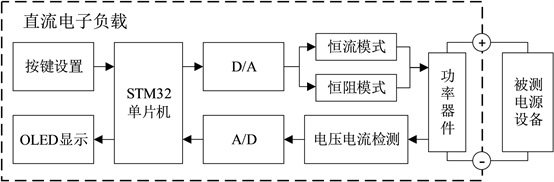Figure 1. Overall system design framework

3. 系统硬件设计

3.1. 直流电子负载主电路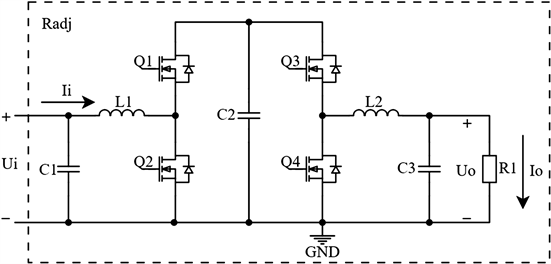Figure 2. Series-connected dual half-bridge DC-DC main circuit topology

${R}_{adj}=\frac{{U}_{i}}{{I}_{i}}$ (1)

${U}_{i}{I}_{i}=\frac{{U}_{i}{}^{2}}{{R}_{adj}}=\frac{{U}_{o}{}^{2}}{{R}_{1}}$ (2)

3.2. 主控单片机的选用

3.3. 驱动电路Figure 3. IR2103 driver circuit

3.4. 采样电路

3.5. 辅助电源Figure 4. INA282 current sampling circuit

4. 系统软件设计

4.1. 软件整体设计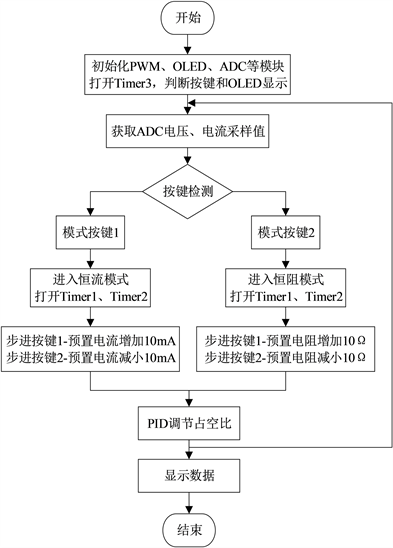Figure 5. Overall framework of software design

BUCK电路，从而调节两部分DC-DC电路的调制比。系统通过定时器Timer3读取按键和OLED显示，采用矩阵键盘设定恒定电流值或恒定电阻值，OLED实时显示系统状态。单片机不断检测与采样端口，一旦检测到信号改变，执行相应的子程序，控制PWM波的占空比，从而实现电子负载的电流或电阻稳定。系统软件设计整体流程图如图5所示。

4.2. PID算法

PID算法控制是设计控制器时最常用、最经典的控制方式，在电气与自控领域有非常广泛的应用 。在稳定电子负载的电流和电阻均使用PID调节控制，在系统闭环控制系统中能发挥稳定作用。它的原理易理解，离散的数据也让计算更加容易，且只需要确认三个参数，积分参数电路控制一般是用不到的，应用最多的是比例微分调节，所以其具有参数选定比较简单的优点。PID控制的基本原理如图6所示。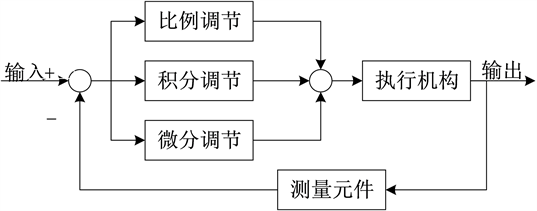Figure 6. PID control schematic

$u\left(k\right)={k}_{p}e\left(k\right)+{k}_{i}\underset{0}{\overset{k}{\sum }}e\left(n\right)+{k}_{d}\left(e\left(k\right)-e\left(k-1\right)\right)$ (3)

4.3. PWM调制技术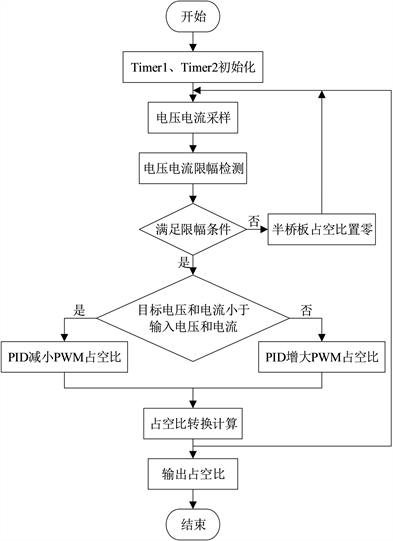Figure 7. PWM modulation algorithm flow chart

5. 测试方案与结果

1) 恒流模式的电流设置范围100~2000 mA，设置分辨率为10 mA，并实时测量和显示流过电子负载的电流，结果见表1Table 1. Constant current mode preset current test records

2) 恒流模式下，当电子负载两端电压变化10 V时，测试电流变化情况，结果见表2Table 2. Constant current mode electronic load voltage change of 10 V when the current change records

3) 恒阻模式的电阻设定范围10~100 Ω，设置分辨率为10 mA，并实时测量和显示电子负载电阻值，结果见表3Table 3. Constant resistance mode preset resistance test records

4) 硬件实物图如图8所示。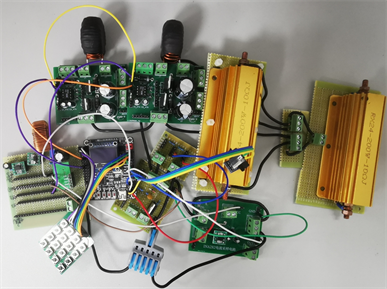Figure 8. Overall circuit physical picture

6. 结论

  夏桂书. 基于STC单片机的智能电子负载[J]. 实验室研究与探索, 2014, 33(3): 115-130.  刘磊. 基于MSP430单片机的直流电子负载设计[J]. 电子科技, 2013, 26(2): 25-27.  王成智. 单相电力电子负载研究与设计[D]: [博士学位论文]. 武汉: 华中科技大学, 2008.  崔建国, 宁永香. 消除密勒电容实现光电耦合器快速工作的研究[J]. 山西电子技术, 2020(4): 91-93.  厉俊, 郑佳蕙. 基于STM32的开关电源模块并联供电系统[J]. 软件工程, 2020, 23(3): 4-8.  徐贵鑫, 许金宇, 王利斌, 金海. 基于STM32的单相不间断式UPS电源设计与实现[J]. 软件工程与应用, 2021, 10(3): 302-310. https://doi.org/10.12677/SEA.2021.103034  张西晨, 徐颖捷, 包齐栋. 基于STM32的简易数控直流稳压电源系统的设计[J]. 软件工程与应用, 2021, 10(3): 354-364. https://doi.org/10.12677/SEA.2021.103040  柳彦钊. 基于STM32的数控开关电源设计[D]: [硕士学位论文]. 西安: 西安科技大学, 2019.